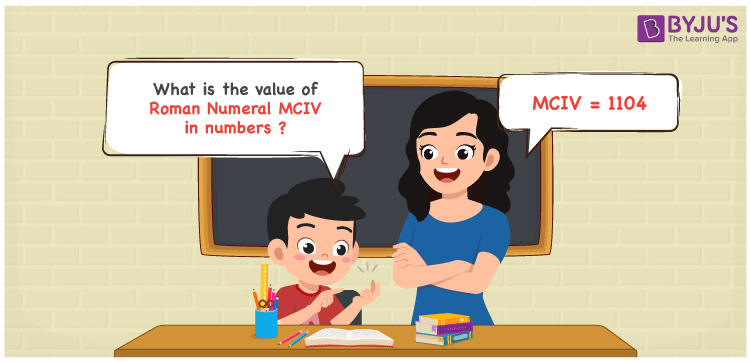Checkout JEE MAINS 2022 Question Paper Analysis : Checkout JEE MAINS 2022 Question Paper Analysis :

# MCIV Roman Numerals

MCIV Roman Numerals is 1104. Roman Numerals are the letters used by the ancient Romans to denote numbers. Solving the problems with the help of Roman Numerals Conversion in PDF format on a regular basis enable students to convert any Roman letters into number form effortlessly. Let us learn the simple procedure of expressing the numerical value of Roman Numeral MCIV with a complete explanation in this article.

 Number Roman Numeral 1104 MCIV

## How to Write MCIV Roman Numerals?The below methods can be used to express the numerical value of MCIV Roman Numerals

First Method:

• Break the Roman Numerals into single letters
• MCIV = M + C + V – I
• Write the numerical value of each letter and add/subtract them
• MCIV = 1000 + 100 + 5 – 1 = 1104

Second Method:

In this method, we consider the groups of Roman Numerals for addition or subtraction

• MCIV = M + C + IV = 1000 + 100 + 4 = 1104
• Thus the numerical value of MCIV Roman Numerals is 1104

## Video Lesson on Roman Numerals## Related Articles

Roman Numerals

Roman Numerals 1 to 50

Roman Numerals 1 to 100

Roman Numerals 1 to 1000

L Roman Numeral

Roman Numeral XXXIX

## Frequently Asked Questions on MCIV Roman Numerals

### What is MCIV Roman Numerals?

MCIV Roman Numerals is 1104.

### Find the sum of MCIV and M Roman Numerals.

MCIV = 1104 and M = 1000. 1104 + 1000 = 2104. Since 2104 = MMCIV. Hence the sum of MCIV and M Roman Numerals is MMCIV.

### How do you read the Roman Numeral MCIV in English?

The Roman Numeral MCIV is 1104 and it is read as One Thousand One Hundred Four in English.